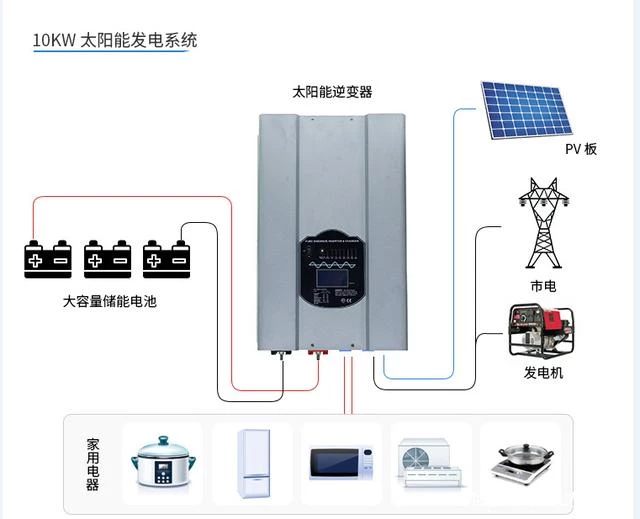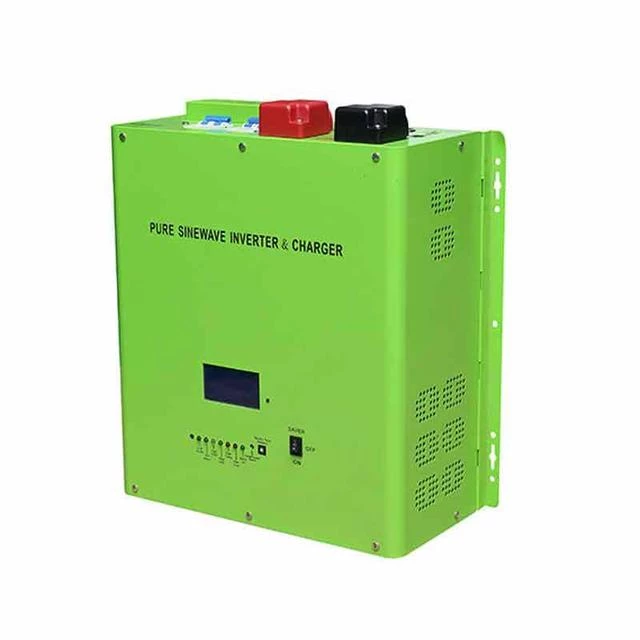Calculation method of solar home system configuration

Calculation method for battery capacity of off-grid solar system
1. Determine the total power of the load, paying attention to the load power, not the rated power of the power supply.
2. Determine the emergency time.
3. Determine the DC voltage level of the power supply.

Calculation method for battery capacity of off-grid solar system
1. Determine the total power of the load, paying attention to the load power, not the rated power of the power supply.
2. Determine the emergency time.
3. Determine the DC voltage level of the power supply.10kw photovoltaic system

Solar photovoltaic system battery capacity method:
1:  Determine the total load power, paying attention to the load power, not the rated power of the power supply;
2:  Determine the emergency time;
3:  Determine the DC voltage level of the power supply; the formula is: (load power/DC voltage)*time*battery coefficient=single cell voltage battery cell number=DC voltage/single cell voltage battery coefficient: 1.6 within half an hour; half From hours to 1 hour is 1.4; more than 1 hour is 1;.For example:
The customer wants a 5KVA UPS, the load is a 3KW light bulb, if you need an emergency for 2 hours, you need to have a battery; the load power is 3000W; the emergency time is 2 hours; the DC voltage level of the UPS is 192V; (3000/192 )*2*1=31.5, find peripheral capacity batteries, you can use 30AH/12V batteries or 33AH/12V batteries; the number of battery cells=192/12V=16; so this solution can use 16 30AH/12V batteries Or 16 batteries of 33AH/12V; For DC screens and communication power supplies, customers usually directly say how many A systems or how many AH systems; 100AH means that 100A can be discharged within 1 hour, but the battery discharge coefficient should be considered; It is too short to actually discharge 100A; but a 10-hour discharge rate battery can discharge 10A per hour, which can be discharged for 10 hours;